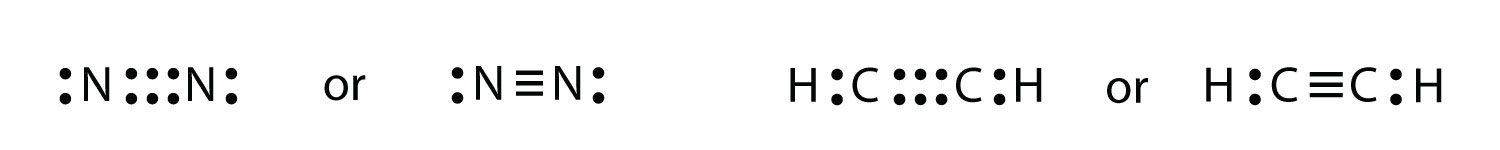# 12.06: Lewis Structures

There is a simple set of steps for determining the Lewis electron dot diagram of a simple molecule. First, you must identify the central atom and the surrounding atoms. The central atom is the atom in the center of the molecule, while the surrounding atoms are the atoms making bonds to the central atom. The central atom is usually written first in the formula of the compound (H2O is the notable exception). After the central and surrounding atoms have been identified, follow these steps:

1. Count the total number of valence electrons. Add extra if the species has negative charges and remove some for every positive charge on the species.
2. Write the central atom and surround it with the surrounding atoms.
3. Put a pair of electrons between the central atom and each surrounding atom.
4. Complete the octets around the surrounding atoms (except for H).
5. Put remaining electrons, if any, around the central atom.
6. Check that every atom has a full valence shell.

Let us try these steps to determine the electron dot diagram for BF4. The B atom is the central atom, and the F atoms are the surrounding atoms. There is a negative sign on the species, so we have an extra electron to consider.

1. Count the total number of electrons. B has 3, each F has 7, and there is one extra electron: 3 + 7 + 7 + 7 + 7 + 1 = 32.

2. Write the central atom surrounded by surrounding atoms.3. Put a pair of electrons between the central atom and each surrounding atom. This uses up eight electrons, so we have 32 − 8 = 24 electrons left.4. Complete the octets around the surrounding atoms (except for H). This uses up 24 more electrons, leaving 24 − 24 = 0 electrons left.5. Put remaining electrons, if any, around the central atom. There are no additional electrons to add to the central atom.

6. Check. The B atom has eight electrons around it, as does each F atom. Each atom has a complete octet. This is a good Lewis electron dot diagram for BF4.

Sometimes, however, these steps do not work. If we were to follow these steps for the compound formaldehyde (CH2O), we would get the following:The H and O atoms have the proper number of electrons, but the C atom has only six electrons around it, not the eight electrons for an octet. How do we fix this?

We fix this by recognizing that two atoms can share more than one pair of electrons. In the case of CH2O, the O and C atoms share two pairs of electrons, with the following Lewis electron dot diagram as a result:By circling the electrons around each atom, we can now see that the O and C atoms have octets, while each H atom has two electrons:Each valence shell is full, so this is an acceptable Lewis electron dot diagram. If we were to use lines to represent the bonds, we would use two lines between the C and O atoms:The bond between the C and O atoms is a double bond and represents two bonding pairs of electrons between the atoms. If using the rules for drawing Lewis electron dot diagrams do not work as written, a double bond may be required.

Example $$\PageIndex{3}$$: Carbon Dioxide

What is the proper Lewis electron dot diagram for CO2?

Solution

The central atom is a C atom, with O atoms as surrounding atoms. We have a total of 4 + 6 + 6 = 16 valence electrons. Following the rules for Lewis electron dot diagrams for compounds gives usThe O atoms have complete octets around them, but the C atom has only four electrons around it. The way to solve this dilemma is to make a double bond between carbon and each O atom:Each O atom still has eight electrons around it, but now the C atom also has a complete octet. This is an acceptable Lewis electron dot diagram for CO2.

Exercise $$\PageIndex{3}$$

What is the proper Lewis electron dot diagram for carbonyl sulfide (COS)?It is also possible to have a triple bond, in which there are three pairs of electrons between two atoms. Good examples of this are elemental nitrogen (N2) and acetylene (C2H2):Acetylene is an interesting example of a molecule with two central atoms, which are both C atoms. Polyatomic ions are bonded together with covalent bonds. Because they are ions, however, they participate in ionic bonding with other ions. So both major types of bonding can occur at the same time.

## Summary

• Covalent bonds are formed when atoms share electrons.
• Lewis electron dot diagrams can be drawn to illustrate covalent bond formation.
• Double bonds or triple bonds between atoms may be necessary to properly illustrate the bonding in some molecules.